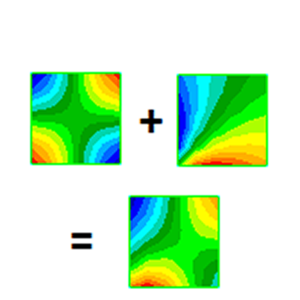WRITTEN BY:

Introduction

Superposition is used in many different scenarios for structural calculations. In fact, the method is so useful that multiple names were even “invented” for it when used for a specific application: Static load combinations. Modal dynamics. Load-case combinations. Load reconstruction.

Because so many different methods use it, a separate article on the topic is written instead of repeating it for forthcoming blog articles on some of the methods that use it.

In this shorter article, we will briefly look at the assumptions and mathematics behind superposition, as well as some of the very useful implications for the method.

The (very simple) mathematics behind Superposition

It all starts with a linear system with a matrix equation of the form: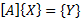In this,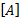is a matrix while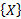and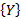are vectors.

Before you comment on the vectors: Yes, the vectors can be replaced with matrices and superposition will still hold. The reason for this choice of starting point is simply because it is an extremely common equation in FEA. By replacing the vectors with matrices all the equations will still hold.

Let’s defineas: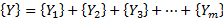Correspondingly, we’ll define a set ofwithvarying from 1 to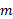such that: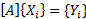We can now combine the previous two equations: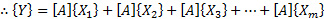This can be rearranged as: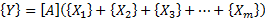Comparing this with the first equation, we find that: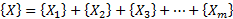And that is all there is to superposition!

Unfortunately, it isn’t obvious what we just obtained, so this brings us to the meaning of superposition

What is superposition used for?

The easiest to explain the above is to use an example: Let’s take the example of the linear static equation: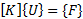In this case,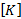,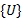and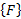are respectively the stiffness matrix, displacement vector, and applied load.

Now let us apply multiple loads to the structure, but we apply the loads one at a time. For each load we will obtain a different displacement state: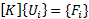To solve the displacement state due to all loads simultaneously, we can solve the equation again with a load vector containing all the loads.

However, we don’t need to because of the superposition principle which says that for a system:Whereis defined as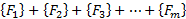, and for which we already have the displacements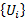for every one of the loads, then we can simply add allto find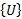: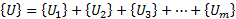This means that it is possible to split a complex load into simpler ones to find the effect of each. Then we can combine the results of these simpler loads to find the result due to them combined.

Scaling results

It gets better: Since we have a linear system, if a load is scaled by a scalar number then the result scales by the same amount. To see why start with a linear system:Multiply each side by a scalar value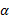: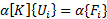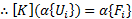Therefore, for a load of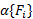, the displacement is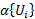. In other words, 10x the load causes 10x the displacement.

How is this better? This means that we don’t need to know the magnitude of the load beforehand as we can independently scale the result of each of the loads that make up the total applied load.

Practical use of superposition

Linear statics

Lots of structures require analysis of multiple load combinations.

An example is a slowly rotating structure under gravity load. This can be represented statically by “rotating” the gravity vector.

If we don’t use superposition, we would run a lot of load-cases, each with gravity at a different angle. If our angular step-size between load-cases is not small enough, it is easy to miss the exact angle with the worst stress state.

Using superposition makes the simulation a lot easier and allows the stress state to be viewed at any angle. The superposition requires only two load-cases to be analyzed: The first with the gravity load where the structure is at zero degrees, the second when it has rotated by 90 degrees. In other words, one with gravity pointing down, the other with gravity pointing sideways. This is possible because any other angle is simply a linear combination of these two, each scaled by the correct amount to represent the gravity load at the angle of interest.

This is the same calculations we needed to make for the original model (without superposition) to find the gravity magnitude and direction for each load case. The only difference is that we do it as a post-processing step instead of having to run an analysis with a lot of different load-angles.

Other examples are for structures with varying loads under operation. Not all loads are active at the same time, so we set up a list of which loads (each with a corresponding scale factor) are possible. Then we can combine the results in the post-processor to find the worst combination of loads.

Modal dynamics

In modal dynamics, we assume that the deformed shape at any point in time is the sum of the mode shapes, each with a time-dependent scale factor applied. This is discussed in more detail in An introduction to Modal methods for Dynamic Analysis